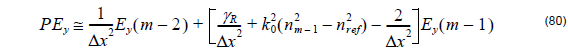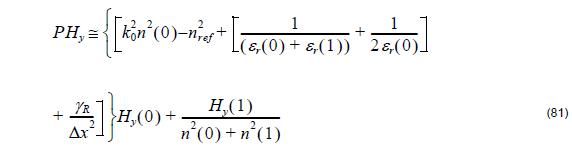We can include the left-hand TBC expressing the field of the hypothetical node φ–1 (see Figure ) outside the analysis domain, (shown in “Transparent Boundary Condition” on page 23), asHere the parameter γL  is determined by the known fields at z .

Following similar way described we can include the right-hand TBC expressing the field

φ of the hypothetical node asHere, the parameter γis determined by the known fields at z .

Therefore, to include the TBC for TE modes we get:for the first left mesh point =  0 , andfor the last right mesh point.

For TM modes we get:for the first left mesh point i = 0 andfor the last right mesh point i = m -1 .